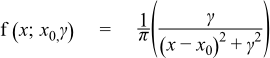#Boost C++ Libraries

...one of the most highly regarded and expertly designed C++ library projects in the world.

This is the documentation for an old version of boost. Click here for the latest Boost documentation.
##### Cauchy-Lorentz Distribution

`#include <boost/math/distributions/cauchy.hpp>`

```template <class RealType = double,
class Policy   = policies::policy<> >
class cauchy_distribution;

typedef cauchy_distribution<> cauchy;

template <class RealType, class Policy>
class cauchy_distribution
{
public:
typedef RealType  value_type;
typedef Policy    policy_type;

cauchy_distribution(RealType location = 0, RealType scale = 1);

RealType location()const;
RealType scale()const;
};
```

The Cauchy-Lorentz distribution is named after Augustin Cauchy and Hendrik Lorentz. It is a continuous probability distribution with probability distribution function PDF given by:The location parameter x0   is the location of the peak of the distribution (the mode of the distribution), while the scale parameter γ   specifies half the width of the PDF at half the maximum height. If the location is zero, and the scale 1, then the result is a standard Cauchy distribution.

The distribution is important in physics as it is the solution to the differential equation describing forced resonance, while in spectroscopy it is the description of the line shape of spectral lines.

The following graph shows how the distributions moves as the location parameter changes:While the following graph shows how the shape (scale) parameter alters the distribution:##### Member Functions
```cauchy_distribution(RealType location = 0, RealType scale = 1);
```

Constructs a Cauchy distribution, with location parameter location and scale parameter scale. When these parameters take their default values (location = 0, scale = 1) then the result is a Standard Cauchy Distribution.

Requires scale > 0, otherwise calls domain_error.

```RealType location()const;
```

Returns the location parameter of the distribution.

```RealType scale()const;
```

Returns the scale parameter of the distribution.

##### Non-member Accessors

All the usual non-member accessor functions that are generic to all distributions are supported: Cumulative Distribution Function, Probability Density Function, Quantile, Hazard Function, Cumulative Hazard Function, mean, median, mode, variance, standard deviation, skewness, kurtosis, kurtosis_excess, range and support.

Note however that the Cauchy distribution does not have a mean, standard deviation, etc. See mathematically undefined function to control whether these should fail to compile with a BOOST_STATIC_ASSERTION_FAILURE, which is the default.

Alternately, the functions mean, standard deviation, variance, skewness, kurtosis and kurtosis_excess will all return a domain_error if called.

The domain of the random variable is [-[max_value], +[min_value]].

##### Accuracy

The Cauchy distribution is implemented in terms of the standard library `tan` and `atan` functions, and as such should have very low error rates.

##### Implementation

In the following table x0 is the location parameter of the distribution, γ   is its scale parameter, x is the random variate, p is the probability and q = 1-p.

Function

Implementation Notes

pdf

Using the relation: pdf = 1 / (π * γ * (1 + ((x - x0 ) / γ)2)

cdf and its complement

The cdf is normally given by:

p = 0.5 + atan(x)/π

But that suffers from cancellation error as x -> -∞. So recall that for `x < 0`:

atan(x) = -π/2 - atan(1/x)

Substituting into the above we get:

p = -atan(1/x) ; x < 0

So the procedure is to calculate the cdf for -fabs(x) using the above formula. Note that to factor in the location and scale parameters you must substitute (x - x0 ) / γ   for x in the above.

This procedure yields the smaller of p and q, so the result may need subtracting from 1 depending on whether we want the complement or not, and whether x is less than x0 or not.

quantile

The same procedure is used irrespective of whether we're starting from the probability or its complement. First the argument p is reduced to the range [-0.5, 0.5], then the relation

x = x0 ± γ   / tan(π * p)

is used to obtain the result. Whether we're adding or subtracting from x0 is determined by whether we're starting from the complement or not.

mode

The location parameter.